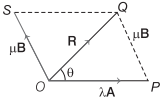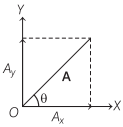# Rotation of a Vector Definition, Formulas – Scalars and Vectors

## Rotation of a Vector Definition, Formulas – Scalars and Vectors

We are giving a detailed and clear sheet on all Physics Notes that are very useful to understand the Basic Physics Concepts.

Rotation of a Vector By an Angle:
(i) If a vector is rotated through an angle θ, which is not an integral multiple of 2π, the vector changes.
(ii) If the frame of reference is rotated or translated, the given vector does not change. The components of the vector may, however, change.

Resolution of Vectors into Two Components
If two-component vectors of R are OP and PQ in the direction of A and B, respectively, and suppose OP = λA and PQ = µB, where λ and µ are two real numbers.Then, resultant vector, R = λA + µB

Resolution of a Vector into its Rectangular ComponentsIf any vector A subtends an angle θ with X-axis, then its
horizontal component, Ax = A cos θ
Vertical component, Ay = A sin θ
Magnitude of vector, A = $$\sqrt{A_{x}^{2}+A_{y}^{2}}$$
tan θ = $$\frac{A_{y}}{A_{x}}$$
Angle, θ = tan-1$$\left(\frac{A_{y}}{A_{x}}\right)$$

Scalars and Vectors Topics: